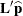# The MIXED Procedure

### ESTIMATE Statement

• ESTIMATE ’label’ <fixed-effect values …> <|random-effect values …> </ options>;

The ESTIMATE statement is exactly like a CONTRAST statement, except only one-rowmatrices are permitted. The actual estimate,, is displayed along with its approximate standard error. An approximate t test that= 0 is also produced.

PROC MIXED selects the degrees of freedom to match those displayed in the "Tests of Fixed Effects" table for the final effect you list in the ESTIMATE statement. You can modify the degrees of freedom by using the DF= option.

If PROC MIXED finds the fixed-effects portion of the specified estimate to be nonestimable, then it displays "Non-est" for the estimate entries.

The following examples of ESTIMATE statements compute the mean of the first level of `A` in the split-plot example (see Example 65.1) for various inference spaces:

```estimate 'A1 mean narrow'   intercept 1
A 1 B .5 .5 A*B .5 .5 |
block   .25 .25 .25 .25
A*Block .25 .25 .25 .25
0   0   0   0
0   0   0   0;
estimate 'A1 mean intermed' intercept 1
A 1 B .5 .5 A*B .5 .5 |
Block .25 .25 .25 .25;
estimate 'A1 mean broad'    intercept 1
A 1 B .5 .5 A*B .5 .5;
```

The construction of thevector for an ESTIMATE statement follows the same rules as listed under the CONTRAST statement.

Table 65.5 summarizes the options available in the ESTIMATE statement.

Table 65.5: ESTIMATE Statement Options

Option

Description

Specifies the confidence level

Constructs t-type confidence limits

Specifies the degrees of freedom

Specifies a value by which to divide all coefficients

Displays thematrix coefficients

Sets up random-effect contrasts between different groups

Performs lower-tailed tests

Tunes the estimability checking

Sets up random-effect contrasts between different subjects

Performs upper-tailed tests

You can specify the following options in the ESTIMATE statement after a slash (/).

ALPHA=number

requests that a t-type confidence interval be constructed with confidence level 1 – number. The value of number must be between 0 and 1; the default is 0.05.

CL

requests that t-type confidence limits be constructed. The confidence level is 0.95 by default; this can be changed with the ALPHA= option.

DF=number

specifies the degrees of freedom for the t test and confidence limits. The default is the denominator degrees of freedom taken from the "Tests of Fixed Effects" table and corresponds to the final effect you list in the ESTIMATE statement.

DIVISOR=number

specifies a value by which to divide all coefficients so that fractional coefficients can be entered as integer numerators.

E

requests that thematrix coefficients be displayed. The ODS name of this "L Matrix Coefficients" table is "Coef."

GROUP coeffs
GRP coeffs

sets up random-effect contrasts between different groups when a GROUP= variable appears in the RANDOM statement. By default, ESTIMATE statement coefficients on random effects are distributed equally across groups.

LOWER
LOWERTAILED

requests that the p-value for the t test be based only on values less than the t statistic. A two-tailed test is the default. A lower-tailed confidence limit is also produced if you specify the CL option.

SINGULAR=number

tunes the estimability checking as documented for the SINGULAR= option in the CONTRAST statement.

SUBJECT coeffs
SUB coeffs

sets up random-effect contrasts between different subjects when a SUBJECT= variable appears in the RANDOM statement. By default, ESTIMATE statement coefficients on random effects are distributed equally across subjects. For example, the ESTIMATE statement in the following code from Example 65.5 constructs the difference between the random slopes of the first two batches.

```proc mixed data=rc;
class batch;
model y = month / s;
random int month / type=un sub=batch s;
estimate 'slope b1 - slope b2' | month 1 / subject 1 -1;
run;
```
UPPER
UPPERTAILED

requests that the p-value for the t test be based only on values greater than the t statistic. A two-tailed test is the default. An upper-tailed confidence limit is also produced if you specify the CL option.